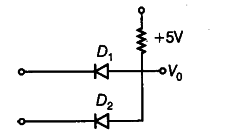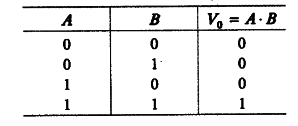# Write the truth table for the circuit shown in figure below. Name the gate that the circuit resembles

Write the truth table for the circuit shown in figure below. Name the gate that the circuit resembles.The circuit resembles AND gate. The Boolean expression of this circuit is, { V }_{ 0 } = A-B i.e. { V }_{ 0 } equals A AND B. The truth table of this gate is as given below: